Below are some typical calculations and formulas.  Some of these are from references and some are from Wikepedia.  I put these together for my theory.  You will realize that a relatively massive micro black hole ball or string of entropy is way too small to suck in the Earth, especially when it is triggering low energy nuclear reactions at its surface/firewall and creating a coma of gas and transmuted material around it.   This is a work in progress and I will put a link on the menu to these so it will always have the latest.

Typical Solar Coronal Mass Ejection Mass:

Coronal mass ejections reach velocities between 20km/s to 3200km/s with an average speed of 489km/s, based on SOHO/LASCO measurements between 1996 and 2003. The average mass is 1.6×1012kg.

1) Typical Micro Black Hole Calculations.  I believe our “Weather Particles” are micro black hole balls and strings of entropy with varying energy levels.  Their “Mass” reacts gravitationally (entropically) but they are really just a ball of energy (entropy).  1e+10 kg is less than 1% of an average coronal mass ejection mass.

 Parameter Value Units Expression Mass 1.000000e+10 kilogramsRadius 1.484852e-17 metersSurface area 2.770613e-33 sq. metersSurface gravity 3.086084e+32 Earth gravitiesSurface tides 4.076386e+50 meter/second2/meterEntropy 1.151841e+36 –Temperature 1.227203e+13 kelvinLuminosity 3.563442e+12 wattsLifetime 2664132 years## Typical Particle Orbit Calculations

Kepler 2-body Elliptical  (ie. a double rainbow particle with another larger particle)

Assume:

where:

•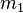andare the masses of the two bodies.
Then:

for any non-negative e, called the eccentricity• the positions of the bodies with respect to the barycenter are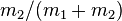andtimes r, respectively, so the two bodies’ orbits are similar conic sections; the same ratios apply for the velocities, and, without the minus, for the angular momentum and for the kinetic energies, all with respect to the barycenter
• for circular orbits• for elliptic orbits(with a expressed in AU and T in years, and with M the total mass relative to that of the Sun, we get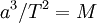)
• for parabolic trajectoriesis constant and equal to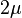• the formulas for the specific orbital energyapply, with specific potential and kinetic energy and their sum taken as the totals for the system, divided by the reduced mass; the kinetic energy of the smaller body is larger; the potential energy of the whole system is equal to the potential energy of one body with respect to the other, i.e. minus the energy needed to escape the other if the other is kept in a fixed position; this should not be confused with the smaller amount of energy one body needs to escape, if the other body moves away also, in the opposite direction: in that case the total energy the two need to escape each other is the same as the aforementioned amount; the conservation of energy for each mass means that an increase of kinetic energy is accompanied by a decrease of potential energy, which is for each mass the inner product of the force and the change in position relative to the barycenter, not relative to the other mass
• for elliptic and hyperbolic orbitsMulti-Body

As I have researched, it is very clear to me that these quantum “weather” particles are following multi-body orbits, since there appear to be many orbiting through entangled jet streams concurrently. This is the most elemental way of solving the n-body problem, the theoretical expression. It is often called “The n-body problem by Taylor series“, which is an implementation of the Power series solution of differential equations.

We start by defining the differential equations system:where (in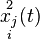) the upper indexindicates the second derivative with respect to timerepresents the number of each body andthe coordinate.

Because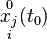and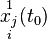are given as initial conditions, then every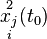are known. Doing implicit derivation over everyresults inwhich atare known because each depends on knownprecalculated and given constants and then the Taylor series are constructed theoretically in such way, performing this process infinitely.

Beta Decay (At the surface of the particles)

## β− decay

In β− decay, the weak interaction converts an atomic nucleus into a nucleus with one higher atomic number while emitting an electron (e−) and an electron antineutrino (ν
e):

A
ZN → A
Z+1N’ + e− + ν


where A and Z are the mass number and atomic number of the decaying nucleus. For example, the free neutron (1
0n) decays by β− decay into a proton (p):

n → p + e− + ν
e.

At the fundamental level (as depicted in the Feynman diagram on the right), this is caused by the conversion of the negatively charged down quark to the positively charged up quark by emission of a W− boson; the W− boson subsequently decays into an electron and an electron antineutrino:

d → u + e− + ν
e.

β− decay generally occurs in neutron-rich nuclei.

## β+ decay

In β+ decay, or “positron emission” (sometimes considered to be a type of beta decay), the weak interaction converts a nucleus into its next-lower neighbor on the periodic table while emitting a positron (e+) and an electron neutrino (ν
e):

A
ZN → A
Z−1N’ + e+ + ν


β+ decay cannot occur in an isolated proton because it requires energy due to the mass of the neutron being greater than the mass of the proton.β+ decay can only happen inside nuclei when the daughter nucleus has a greater binding energy (and therefore a lower total energy) than the mother nucleus. The difference between these energies goes into the reaction of converting a proton into a neutron, a positron and a neutrino and into the kinetic energy of these particles. In an opposite process to negative beta decay, the weak interaction converts a proton into a neutron by converting an up quark into a down quark by having it emit a W+ or absorb a W−

## Nuclear transmutation

If the proton and neutron are part of an atomic nucleus, these decay processes transmute one chemical element into another. For example:

 137 55Cs → 137 56Ba + e− + ν e (beta minus decay) 22 11Na → 22 10Ne + e+ + ν e (beta plus decay) 22 11Na + e− → 22 10Ne + ν e (electron capture)

## Thermonuclear fusion

is a way to achieve nuclear fusion by using extremely high temperatures.

Temperature is a measure of the average kinetic energy of particles, so by heating the material it will gain energy. After reaching sufficient temperature, given by the Lawson criterion, the energy of accidental collisions within the plasma is high enough to overcome the Coulomb barrier and the particles may fuse together.

In a Deuterium – tritium fusion reaction, for example, the energy necessary to overcome the Coulomb barrier is 0.1 MeV. Converting the units between electronvolts and kelvin shows that the barrier would be overcome at a temperature in excess of 120 million kelvins (you will notice the surface temperature of our particle @ 1e+10 kg is hotter than this, thus the source of his energetics).

I also believe that the radiation from the above reactions is redshifted at the surface of the particles due to quantum gravity and so it is of very low energy when it reaches the surroundings, which is very good for us humans.  There has been much recent research on low energy nuclear reactions (LENR) and information can be found here and here.

Earth’s Entropic Dark Matter Dynamo Core

Lower Limit

Estimated Inner Core Mass:                        1.162e+23 kg

Estimated Black Hole Radius:                     0.0001725397 meters

Estimated Black Hole Temperature:        1.056113 Deg. Kelvin

Upper Limit (This may be larger, I think we are missing the mass of the Earth’s magnetic fields which I believe carry considerable mass)

Estimated Inner Core/Outer Core Mass    1.719e+24 kg + 1.162e+23 = 1.8352e+24 kg

Estimated Black Hole Radius:                     0.002725000 meters

Estimated Black Hole Temperature:        0.06687027 Deg. Kelvin

Black Hole Thermodynamics

Bekenstein-Hawking entropy

Thermodynamics of a Black Hole (Borrowed from Wilkipedia)

The four laws of black hole mechanics are physical properties that black holes are believed to satisfy. The laws, analogous to the laws of thermodynamics, were discovered by Brandon Carter,Stephen Hawking and James Bardeen.

### Statement of the laws

The laws of black hole mechanics are expressed in geometrized units.

#### The Zeroth Law

The horizon has constant surface gravity for a stationary black hole.

#### The First Law

Change of mass is related to change of area, angular momentum, and electric charge by:whereis the massis the surface gravity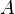is the horizon area,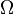is the angular velocityis the angular momentumis the electrostatic potential andis the electric charge.

#### The Second Law

The horizon area is, assuming the weak energy condition, a non-decreasing function of time,This “law” was superseded by Hawking’s discovery that black holes radiate, which causes both the black hole’s mass and the area of its horizon to decrease over time.

#### The Third Law

It is not possible to form a black hole with vanishing surface gravity.= 0 is not possible to achieve.

### Discussion of the laws

#### The Zeroth Law

The zeroth law is analogous to the zeroth law of thermodynamics which states that the temperature is constant throughout a body in thermal equilibrium. It suggests that the surface gravity is analogous to temperatureT constant for thermal equilibrium for a normal system is analogous toconstant over the horizon of a stationary black hole.

#### The First Law

The left hand side, dM, is the change in mass/energy. Although the first term does not have an immediately obvious physical interpretation, the second and third terms on the right hand side represent changes in energy due to rotation and electromagnetism. Analogously, the first law of thermodynamics is a statement of energy conservation, which contains on its right hand side the term T dS.

#### The Second Law

The second law is the statement of Hawking’s area theorem. Analogously, the second law of thermodynamics states that the change in entropy in an isolated system will be greater than or equal to 0 for a spontaneous process, suggesting a link between entropy and the area of a black hole horizon. However, this version violates the second law of thermodynamics by matter losing (its) entropy as it falls in, giving a decrease in entropy. Generalized second law introduced as total entropy = black hole entropy + outside entropy.

#### The Third Law

Extremal black holes have vanishing surface gravity. Stating thatcannot go to zero is analogous to the third law of thermodynamics which states, the entropy of a system at absolute zero is a well-defined constant. This is because a system at zero temperature exists in its ground state. Furthermore, ΔS will reach zero at 0 kelvins, but S itself will also reach zero, at least for perfect crystalline substances. No experimentally verified violations of the laws of thermodynamics are known.

Entropy of a Gas: (Borrowed from NASA – some of my tax money)Schrodinger’ Equation

Much ofthis stuff in our atmosphere/jet streams appears to be oscillating “cosmic” strings of vacuum energy. They will undergo quantum decoherence and decay and follow Schrodinger’s equation, of which there are a few different varieties but it looks like non-stationary “cosmic strings” cover it.

1.•2.